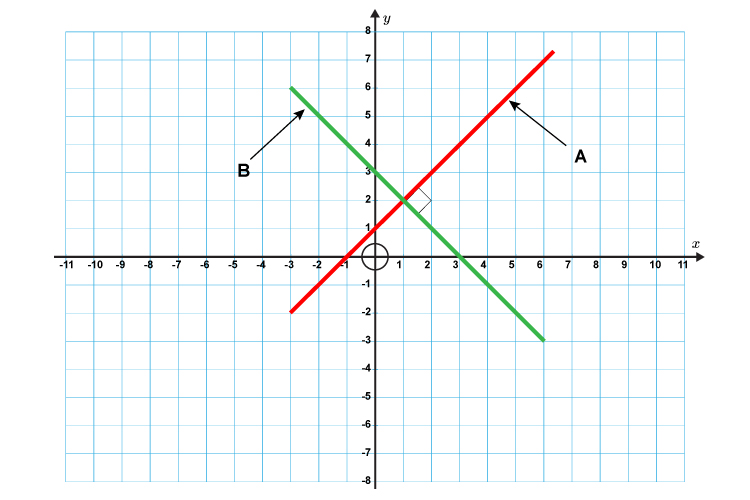Perpendicular lines

m_A\times\m_B=-1

Two lines are perpendicular if you multiply the gradients of the two lines together and the
result is -1.

To remember this formula work out what the slopes of an X would be.Gradient (m) =y/x

Gradient\ of\ li\n\e\ A=1/1=1

Gradient\ of\ li\n\e\ B=1/-1=-1

This shows when we multiply them together 1\times-1 = -1

Therefore m_A\timesm_B=-1